# NCERT Solutions Exercise 3.1: Matrices Notes | Study Mathematics (Maths) Class 12 - JEE

## JEE: NCERT Solutions Exercise 3.1: Matrices Notes | Study Mathematics (Maths) Class 12 - JEE

The document NCERT Solutions Exercise 3.1: Matrices Notes | Study Mathematics (Maths) Class 12 - JEE is a part of the JEE Course Mathematics (Maths) Class 12.
All you need of JEE at this link: JEE

Exercise 3.1

Question 1: In the matrix A=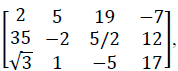write:
(i)   The order of the matrix
(ii)  The number of elements,
(iii) Write the elements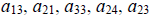(i) In the given matrix, the number of rows is 3 and the number of columns is 4.
Therefore, the order of the matrix is 3 × 4.

(ii)  Since the order of the matrix is 3 × 4, there are 3 × 4 = 12 elements in it.

(iii)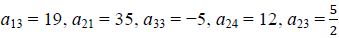Question 2: If a matrix has 24 elements, what are the possible order it can have? What, if it has 13 elements?
We know that if a matrix is of the order m × n, it has mn elements. Thus, to find all the possible orders of a matrix having 24 elements, we have to find all the ordered pairs of natural numbers whose product is 24. The ordered pairs are: (1, 24), (24, 1), (2, 12), (12, 2), (3, 8), (8, 3), (4, 6), and (6, 4)

Hence, the possible orders of a matrix having 24 elements are:

1 × 24, 24 × 1, 2 × 12, 12 × 2, 3 × 8, 8 × 3, 4 × 6, and 6 × 4 (1, 13) and (13, 1) are the ordered pairs of natural numbers whose product is 13.

Hence, the possible orders of a matrix having 13 elements are 1 × 13 and 13 × 1.

Question 3: If a matrix has 18 elements, what are the possible orders it can have? What, if it has 5 elements?
We know that if a matrix is of the order m × n, it has mn elements. Thus, to find all the possible orders of a matrix having 18 elements, we have to find all the ordered pairs of natural numbers whose product is 18.

The ordered pairs are: (1, 18), (18, 1), (2, 9), (9, 2), (3, 6,), and (6, 3)

Hence, the possible orders of a matrix having 18 elements are: 1 × 18, 18 × 1, 2 × 9, 9 × 2, 3 × 6, and 6 × 3 (1, 5) and (5, 1) are the ordered pairs of natural numbers whose product is 5.

Hence, the possible orders of a matrix having 5 elements are 1 × 5 and 5 × 1.

Question 4: Construct a 2 × 2 matrix, A = [aij], whose elements are given by:
1.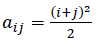2.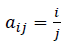3.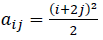(i) Given that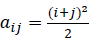In general a 2 × 2 matrix is given by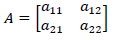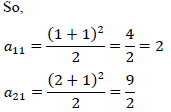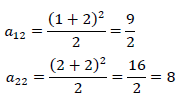Therefore, the required matrix is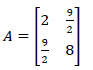(ii) aij=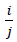In general a 2 × 2 matrix is given by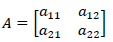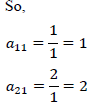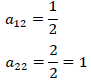Therefore, the required matrix is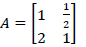(iii) Given that aij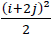In general a 2 × 2 matrix is given by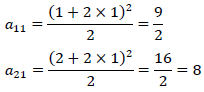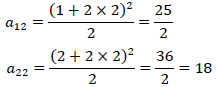Therefore, the required matrix is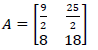Question 5: Construct a 3 × 4 matrix, whose elements are given by
(i) aij =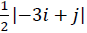(ii) aij =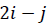(i) aij =In general a 3 × 4 matrix is given by aij =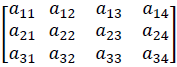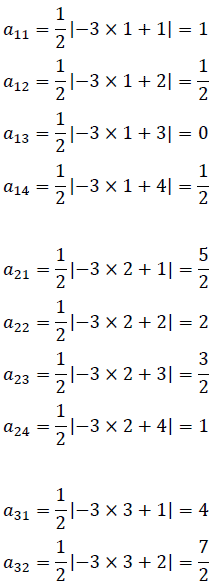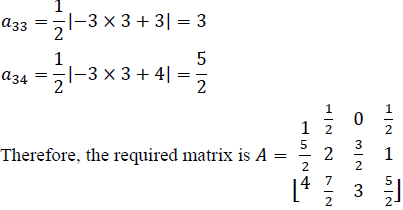(ii) aij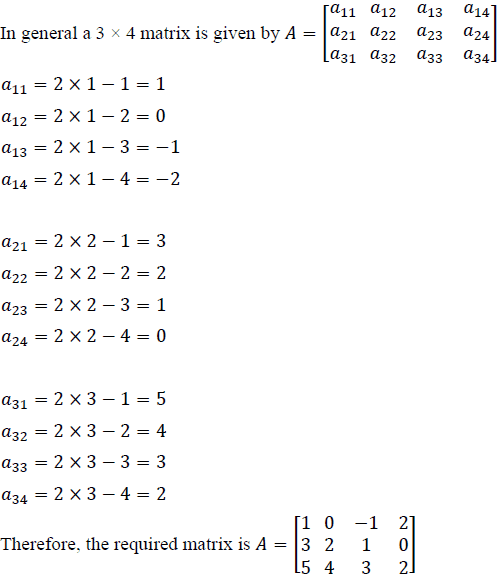Question 6: Find the value of x, y, and z from the following equation:
(i)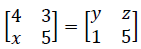(ii)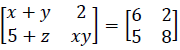(iii)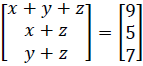(i)As the given matrices are equal, their corresponding elements are also equal.

Comparing the corresponding elements, we get:
x = 1, y = 4, and z = 3

(ii)As the given matrices are equal, their corresponding elements are also equal.
Comparing the corresponding elements, we get:

x + y = 6,

xy = 8,

5 + z = 5
Now, 5 + z = 5 ⇒ z = 0 we know that:
(x − y)2 = (x + y)2 − 4xy
⇒(x − y)2 = 36 − 32 = 4
⇒x − y = ±2
Now, when x − y = 2 and x + y = 6, we get x = 4 and y = 2
When x − y = − 2 and x + y = 6, we get x = 2 and y = 4
∴ x = 4, y = 2, and z = 0 or x = 2, y = 4, and z = 0

(iii)As the two matrices are equal, their corresponding elements are also equal.
Comparing the corresponding elements, we get:
x + y + z = 9 … (1)
x + z = 5 …….. (2)
y + z = 7 …….. (3)
From (1) and (2), we have:
y + 5 = 9
⇒y = 4
Then, from (3), we have:
4 + z = 7
⇒z = 3
∴ x + z = 5
⇒x = 2
∴ x = 2, y = 4 and z = 3.

Question 7: Find the value of a, b, c, and d from the equation: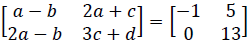As the two matrices are equal, their corresponding elements are also equal.
Comparing the corresponding elements, we get:
a − b = −1 …… (1)
2a − b = 0 …… (2)
2a + c = 5 ……. (3)
3c + d = 13 ….. (4)
From (2), we have:
b = 2a
Then, from (1), we have:
a − 2a = −1

⇒ a = 1

⇒b = 2
Now, from (3), we have:
2 ×1 + c = 5 ⇒ c = 3
From (4) we have:
3 × 3 + d = 13
⇒9 + d = 13

⇒d = 4
∴ a = 1, b = 2, c = 3 and d = 4.

Question 8: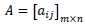is a square matrix, if
(A) m < n
(B) m > n
(C) m = n
(D) None of these
It is known that a given matrix is said to be a square matrix if the number of rows is equal to the number of columns. Therefore,is a square matrix, if m = n.

Question 9: Which of the given values of x and y make the following pair of matrices equal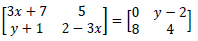(A)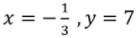(B) Not possible to find
(C)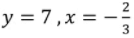(D)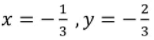It is given that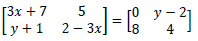Equating the corresponding elements, we get:

3x+7=0 ⇒x = -7/3

and 5=y−2 ⇒y=7

y+1=8 ⇒ y = 7

and 2−3x=4 ⇒x = -2/3

We find that on comparing the corresponding elements of the two matrices, we get two different values of x, which is not possible. Hence, it is not possible to find the values of x and y for which the given matrices are equal.

Question 10: The number of all possible matrices of order 3 × 3 with each entry 0 or 1 is:
(A)27
(B)18
(C)81
(D)512
The given matrix of the order 3 × 3 has 9 elements and each of these elements can be either 0 or 1.
Now, each of the 9 elements can be filled in two possible ways.
Therefore, by the multiplication principle, the required number of possible matrices is 29 = 512.

The document NCERT Solutions Exercise 3.1: Matrices Notes | Study Mathematics (Maths) Class 12 - JEE is a part of the JEE Course Mathematics (Maths) Class 12.
All you need of JEE at this link: JEEUse Code STAYHOME200 and get INR 200 additional OFF

## Mathematics (Maths) Class 12

209 videos|209 docs|139 tests

Track your progress, build streaks, highlight & save important lessons and more!

,

,

,

,

,

,

,

,

,

,

,

,

,

,

,

,

,

,

,

,

,

;